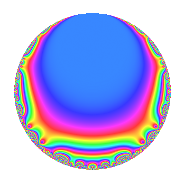# Properties

 Label 4005.2.a.hLevel 4005 Weight 2 Character orbit 4005.a Self dual Yes Analytic conductor 31.980 Analytic rank 0 Dimension 2 CM No Inner twists 1

# Related objects

## Newspace parameters

 Level: $$N$$ = $$4005 = 3^{2} \cdot 5 \cdot 89$$ Weight: $$k$$ = $$2$$ Character orbit: $$[\chi]$$ = 4005.a (trivial)

## Newform invariants

 Self dual: Yes Analytic conductor: $$31.9800860095$$ Analytic rank: $$0$$ Dimension: $$2$$ Coefficient field: $$\Q(\sqrt{5})$$ Coefficient ring: $$\Z[a_1, \ldots, a_{7}]$$ Coefficient ring index: $$2$$ Fricke sign: $$-1$$ Sato-Tate group: $\mathrm{SU}(2)$

## $q$-expansion

Coefficients of the $$q$$-expansion are expressed in terms of $$\beta = \sqrt{5}$$. We also show the integral $$q$$-expansion of the trace form.

 $$f(q)$$ $$=$$ $$q$$ $$+ q^{2}$$ $$- q^{4}$$ $$+ q^{5}$$ $$+ ( 1 + \beta ) q^{7}$$ $$-3 q^{8}$$ $$+O(q^{10})$$ $$q$$ $$+ q^{2}$$ $$- q^{4}$$ $$+ q^{5}$$ $$+ ( 1 + \beta ) q^{7}$$ $$-3 q^{8}$$ $$+ q^{10}$$ $$+ ( 2 + 2 \beta ) q^{11}$$ $$+ ( 2 + 2 \beta ) q^{13}$$ $$+ ( 1 + \beta ) q^{14}$$ $$- q^{16}$$ $$+ 2 q^{17}$$ $$+ ( -5 + \beta ) q^{19}$$ $$- q^{20}$$ $$+ ( 2 + 2 \beta ) q^{22}$$ $$+ ( 3 - \beta ) q^{23}$$ $$+ q^{25}$$ $$+ ( 2 + 2 \beta ) q^{26}$$ $$+ ( -1 - \beta ) q^{28}$$ $$-2 \beta q^{29}$$ $$+ ( 1 - \beta ) q^{31}$$ $$+ 5 q^{32}$$ $$+ 2 q^{34}$$ $$+ ( 1 + \beta ) q^{35}$$ $$+ ( -2 - 2 \beta ) q^{37}$$ $$+ ( -5 + \beta ) q^{38}$$ $$-3 q^{40}$$ $$+ 10 q^{41}$$ $$+ ( 3 - \beta ) q^{43}$$ $$+ ( -2 - 2 \beta ) q^{44}$$ $$+ ( 3 - \beta ) q^{46}$$ $$-8 q^{47}$$ $$+ ( -1 + 2 \beta ) q^{49}$$ $$+ q^{50}$$ $$+ ( -2 - 2 \beta ) q^{52}$$ $$-2 q^{53}$$ $$+ ( 2 + 2 \beta ) q^{55}$$ $$+ ( -3 - 3 \beta ) q^{56}$$ $$-2 \beta q^{58}$$ $$+ ( 1 - \beta ) q^{59}$$ $$+ ( -4 - 2 \beta ) q^{61}$$ $$+ ( 1 - \beta ) q^{62}$$ $$+ 7 q^{64}$$ $$+ ( 2 + 2 \beta ) q^{65}$$ $$+ ( 2 - 2 \beta ) q^{67}$$ $$-2 q^{68}$$ $$+ ( 1 + \beta ) q^{70}$$ $$+ ( 10 - 2 \beta ) q^{71}$$ $$+ 2 q^{73}$$ $$+ ( -2 - 2 \beta ) q^{74}$$ $$+ ( 5 - \beta ) q^{76}$$ $$+ ( 12 + 4 \beta ) q^{77}$$ $$+ ( 4 - 4 \beta ) q^{79}$$ $$- q^{80}$$ $$+ 10 q^{82}$$ $$+ ( 13 + \beta ) q^{83}$$ $$+ 2 q^{85}$$ $$+ ( 3 - \beta ) q^{86}$$ $$+ ( -6 - 6 \beta ) q^{88}$$ $$+ q^{89}$$ $$+ ( 12 + 4 \beta ) q^{91}$$ $$+ ( -3 + \beta ) q^{92}$$ $$-8 q^{94}$$ $$+ ( -5 + \beta ) q^{95}$$ $$-6 q^{97}$$ $$+ ( -1 + 2 \beta ) q^{98}$$ $$+O(q^{100})$$ $$\operatorname{Tr}(f)(q)$$ $$=$$ $$2q$$ $$\mathstrut +\mathstrut 2q^{2}$$ $$\mathstrut -\mathstrut 2q^{4}$$ $$\mathstrut +\mathstrut 2q^{5}$$ $$\mathstrut +\mathstrut 2q^{7}$$ $$\mathstrut -\mathstrut 6q^{8}$$ $$\mathstrut +\mathstrut O(q^{10})$$ $$2q$$ $$\mathstrut +\mathstrut 2q^{2}$$ $$\mathstrut -\mathstrut 2q^{4}$$ $$\mathstrut +\mathstrut 2q^{5}$$ $$\mathstrut +\mathstrut 2q^{7}$$ $$\mathstrut -\mathstrut 6q^{8}$$ $$\mathstrut +\mathstrut 2q^{10}$$ $$\mathstrut +\mathstrut 4q^{11}$$ $$\mathstrut +\mathstrut 4q^{13}$$ $$\mathstrut +\mathstrut 2q^{14}$$ $$\mathstrut -\mathstrut 2q^{16}$$ $$\mathstrut +\mathstrut 4q^{17}$$ $$\mathstrut -\mathstrut 10q^{19}$$ $$\mathstrut -\mathstrut 2q^{20}$$ $$\mathstrut +\mathstrut 4q^{22}$$ $$\mathstrut +\mathstrut 6q^{23}$$ $$\mathstrut +\mathstrut 2q^{25}$$ $$\mathstrut +\mathstrut 4q^{26}$$ $$\mathstrut -\mathstrut 2q^{28}$$ $$\mathstrut +\mathstrut 2q^{31}$$ $$\mathstrut +\mathstrut 10q^{32}$$ $$\mathstrut +\mathstrut 4q^{34}$$ $$\mathstrut +\mathstrut 2q^{35}$$ $$\mathstrut -\mathstrut 4q^{37}$$ $$\mathstrut -\mathstrut 10q^{38}$$ $$\mathstrut -\mathstrut 6q^{40}$$ $$\mathstrut +\mathstrut 20q^{41}$$ $$\mathstrut +\mathstrut 6q^{43}$$ $$\mathstrut -\mathstrut 4q^{44}$$ $$\mathstrut +\mathstrut 6q^{46}$$ $$\mathstrut -\mathstrut 16q^{47}$$ $$\mathstrut -\mathstrut 2q^{49}$$ $$\mathstrut +\mathstrut 2q^{50}$$ $$\mathstrut -\mathstrut 4q^{52}$$ $$\mathstrut -\mathstrut 4q^{53}$$ $$\mathstrut +\mathstrut 4q^{55}$$ $$\mathstrut -\mathstrut 6q^{56}$$ $$\mathstrut +\mathstrut 2q^{59}$$ $$\mathstrut -\mathstrut 8q^{61}$$ $$\mathstrut +\mathstrut 2q^{62}$$ $$\mathstrut +\mathstrut 14q^{64}$$ $$\mathstrut +\mathstrut 4q^{65}$$ $$\mathstrut +\mathstrut 4q^{67}$$ $$\mathstrut -\mathstrut 4q^{68}$$ $$\mathstrut +\mathstrut 2q^{70}$$ $$\mathstrut +\mathstrut 20q^{71}$$ $$\mathstrut +\mathstrut 4q^{73}$$ $$\mathstrut -\mathstrut 4q^{74}$$ $$\mathstrut +\mathstrut 10q^{76}$$ $$\mathstrut +\mathstrut 24q^{77}$$ $$\mathstrut +\mathstrut 8q^{79}$$ $$\mathstrut -\mathstrut 2q^{80}$$ $$\mathstrut +\mathstrut 20q^{82}$$ $$\mathstrut +\mathstrut 26q^{83}$$ $$\mathstrut +\mathstrut 4q^{85}$$ $$\mathstrut +\mathstrut 6q^{86}$$ $$\mathstrut -\mathstrut 12q^{88}$$ $$\mathstrut +\mathstrut 2q^{89}$$ $$\mathstrut +\mathstrut 24q^{91}$$ $$\mathstrut -\mathstrut 6q^{92}$$ $$\mathstrut -\mathstrut 16q^{94}$$ $$\mathstrut -\mathstrut 10q^{95}$$ $$\mathstrut -\mathstrut 12q^{97}$$ $$\mathstrut -\mathstrut 2q^{98}$$ $$\mathstrut +\mathstrut O(q^{100})$$

## Embeddings

For each embedding $$\iota_m$$ of the coefficient field, the values $$\iota_m(a_n)$$ are shown below.

For more information on an embedded modular form you can click on its label.

Label $$\iota_m(\nu)$$ $$a_{2}$$ $$a_{3}$$ $$a_{4}$$ $$a_{5}$$ $$a_{6}$$ $$a_{7}$$ $$a_{8}$$ $$a_{9}$$ $$a_{10}$$
1.1
 −0.618034 1.61803
1.00000 0 −1.00000 1.00000 0 −1.23607 −3.00000 0 1.00000
1.2 1.00000 0 −1.00000 1.00000 0 3.23607 −3.00000 0 1.00000
 $$n$$: e.g. 2-40 or 990-1000 Significant digits: Format: Complex embeddings Normalized embeddings Satake parameters Satake angles

## Inner twists

This newform does not admit any (nontrivial) inner twists.

## Atkin-Lehner signs

$$p$$ Sign
$$3$$ $$-1$$
$$5$$ $$-1$$
$$89$$ $$-1$$

## Hecke kernels

This newform can be constructed as the intersection of the kernels of the following linear operators acting on $$S_{2}^{\mathrm{new}}(\Gamma_0(4005))$$:

 $$T_{2}$$ $$\mathstrut -\mathstrut 1$$ $$T_{7}^{2}$$ $$\mathstrut -\mathstrut 2 T_{7}$$ $$\mathstrut -\mathstrut 4$$ $$T_{11}^{2}$$ $$\mathstrut -\mathstrut 4 T_{11}$$ $$\mathstrut -\mathstrut 16$$